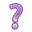## 9.5 Wald test (General F)

The Wald test is used for simultaneous tests of $$Q$$ variables in a model. This is used primarily in two situations:

1. Testing if a categorical variable (with more than 2 levels) as a whole improves model fit
2. Testing a linear combination of predictors (such as a differnece of differences). This topic is not discussed yet

Consider a model with $$P$$ variables and you want to test if $$Q$$ additional variables are useful.

• $$H_{0}: Q$$ additional variables are useless, i.e., their $$\beta$$’s all = 0
• $$H_{A}: Q$$ additional variables are useful to explain/predict $$Y$$

The traditional test statistic that we’ve seen since Intro stats is $$\frac{\hat{\theta}-\theta}{\sqrt{Var(\hat{\theta})}}$$

The Wald test generalizes this test any linear combination of predictors.

$(R\hat{\theta}_{n}-r)^{'}[R({\hat{V}}_{n}/n)R^{'}]^{-1} (R\hat{\theta}_{n}-r) \quad \xrightarrow{\mathcal{D}} \quad F(Q,n-P)$

Where $$\mathbf{R}$$ is the vector of coefficients for the $$\beta$$, and $$\hat{V}_{n}$$ is a consistent estimator of the covariance matrix. Instead of a normal distribution, this test statistic has an $$F$$ distribution with $$Q$$ and $$n-P$$ degrees of freedom.

In the case where we’re testing $$\beta_{p}=\beta_{q}=...=0$$, $$\mathbf{R}$$ is all 1’s.

##### 9.5.0.0.1 Example: Employment status on depression score

Consider a model to predict depression using age, employment status and whether or not the person was chronically ill in the past year as covariates. This example uses the cleaned depression data set.

full_model <- lm(cesd ~ age + chronill + employ, data=depress)
pander(summary(full_model))
Estimate Std. Error t value Pr(>|t|)
(Intercept) 11.48 1.502 7.646 3.191e-13
age -0.133 0.03514 -3.785 0.0001873
chronill 2.688 1.024 2.625 0.009121
employHouseperson 6.75 1.797 3.757 0.0002083
employIn School 1.967 5.995 0.328 0.7431
employOther 4.897 4.278 1.145 0.2533
employPT 3.259 1.472 2.214 0.02765
employRetired 3.233 1.886 1.714 0.08756
employUnemp 7.632 2.339 3.263 0.001238
Fitting linear model: cesd ~ age + chronill + employ
Observations Residual Std. Error $$R^2$$ Adjusted $$R^2$$
294 8.385 0.1217 0.09704

The results of this model show that age and chronic illness are statistically associated with CESD (each p<.006). However employment status shows mixed results. Some employment statuses are significantly different from the reference group, some are not. So overall, is employment status associated with depression?

Recall that employment is a categorical variable, and all the coefficient estimates shown are the effect of being in that income category has on depression compared to being employed full time. For example, the coefficient for PT employment is greater than zero, so they have a higher CESD score compared to someone who is fully employed.

But what about employment status overall? Not all employment categories are significantly different from FT status. To test that employment status affects CESD we need to do a global test that all $$\beta$$’s related to employment status are 0.

$$H_{0}: \beta_{3} = \beta_{4} = \beta_{5} = \beta_{6} = \beta_{7} = \beta_{8} = 0$$
$$H_{A}$$: At least one $$\beta_{j}$$ is not 0.

The regTermTest function can be found in the survey package. The survey package has functions that tend to conflict with dplyr, so it is recommended that you don’t load the package entirely, and use :: notation to call the function directly.

survey::regTermTest(full_model, "employ")
## Wald test for employ
##  in lm(formula = cesd ~ age + chronill + employ, data = depress)
## F =  4.153971  on  6  and  285  df: p= 0.0005092
• Confirm that the degrees of freedom are correct. It should equal the # of categories in the variable you are testing, minus 1.
• Employment has 7 levels, so $$df=6$$.
• Or equivalently, the degrees of freedom are the number of $$beta$$’s you are testing to be 0.

The p-value of this Wald test is significant, thus employment significantly predicts CESD score.What does the vector of coefficients $$R$$ look like here?